Study Materials: ncert solutions

Our ncert solutions for Exercise 10.3 Class 11 maths 10. Straight Lines - ncert solutions - Toppers Study is the best material for English Medium students cbse board and other state boards students.

Solutions ⇒ Class 11th ⇒ Mathematics ⇒ 10. Straight Lines

# Exercise 10.3 Class 11 maths 10. Straight Lines - ncert solutions - Toppers Study

Topper Study classes prepares ncert solutions on practical base problems and comes out with the best result that helps the students and teachers as well as tutors and so many ecademic coaching classes that they need in practical life. Our ncert solutions for Exercise 10.3 Class 11 maths 10. Straight Lines - ncert solutions - Toppers Study is the best material for English Medium students cbse board and other state boards students.

## Exercise 10.3 Class 11 maths 10. Straight Lines - ncert solutions - Toppers Study

CBSE board students who preparing for class 11 ncert solutions maths and Mathematics solved exercise chapter 10. Straight Lines available and this helps in upcoming exams 2023-2024.

### You can Find Mathematics solution Class 11 Chapter 10. Straight Lines

• All Chapter review quick revision notes for chapter 10. Straight Lines Class 11
• NCERT Solutions And Textual questions Answers Class 11 Mathematics
• Extra NCERT Book questions Answers Class 11 Mathematics
• Importatnt key points with additional Assignment and questions bank solved.

NCERT Solutions do not only help you to cover your syllabus but also will give to textual support in exams 2023-2024 to complete exercise 10.3 maths class 11 chapter 10 in english medium. So revise and practice these all cbse study materials like class 11 maths chapter 10.3 in english ncert book. Also ensure to repractice all syllabus within time or before board exams for ncert class 11 maths ex 10.3 in english.

See all solutions for class 11 maths chapter 10 exercise 10 in english medium solved questions with answers.

### Exercise 10.3 class 11 Mathematics Chapter 10. Straight Lines

• Exercise 10.3 Class 11 Maths 10. Straight Lines - Ncert Solutions - Toppers Study
• Class 11 Ncert Solutions
• Solution Chapter 10. Straight Lines Class 11
• Solutions Class 11
• Chapter 10. Straight Lines Exercise 10.3 Class 11

## Exercise 10.3 Class 11 maths 10. Straight Lines - ncert solutions - Toppers Study

Exercise 10.3

Q1. Reduce the following equations into slope - intercept form and find their slopes and the y - intercepts.

(i) x + 7y = 0,

(ii) 6x + 3y – 5 = 0,

(iii) y = 0.

Solution: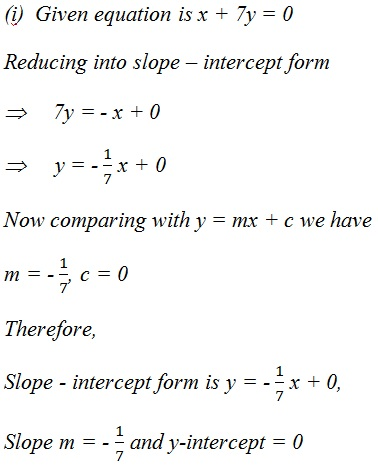Solution: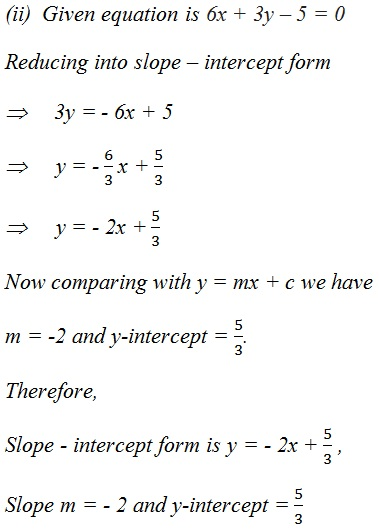Solution:

(iii) y = 0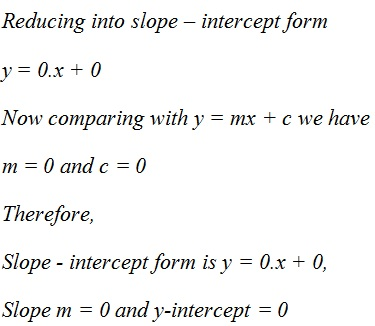Q2. Reduce the following equations into intercept form and find their intercepts on the axes.

(i) 3x + 2y – 12 = 0

(ii) 4x – 3y = 6

(iii) 3y + 2 = 0.

Solution: (i) 3x + 2y – 12 = 0

Reducing into intercept form

3x + 2y = 12

Dividing by 12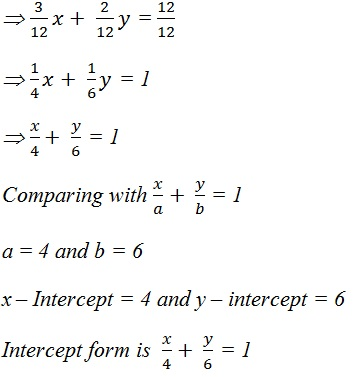Solution: (ii) 4x – 3y = 6

Reducing equation into intercept form

4x – 3y = 6

Dividing by 6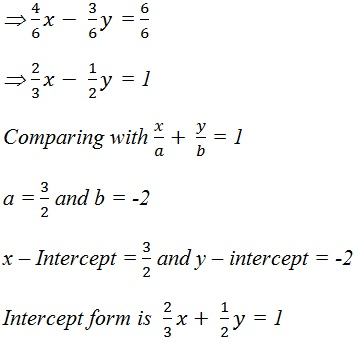Solution: (iii) 3y + 2 = 0

Reducing equation into intercept form

0.x  + 3y  = - 2

Dividing by -2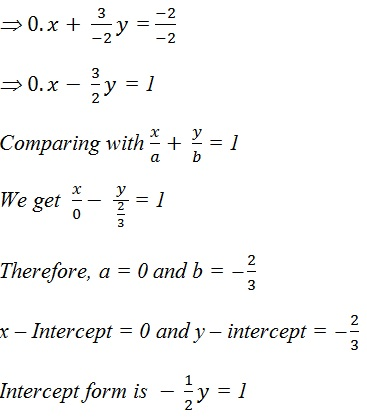Q3. Reduce the following equations into normal form. Find their perpendicular distances from the origin and angle between perpendicular and the positive x-axis.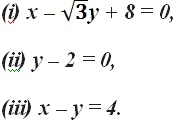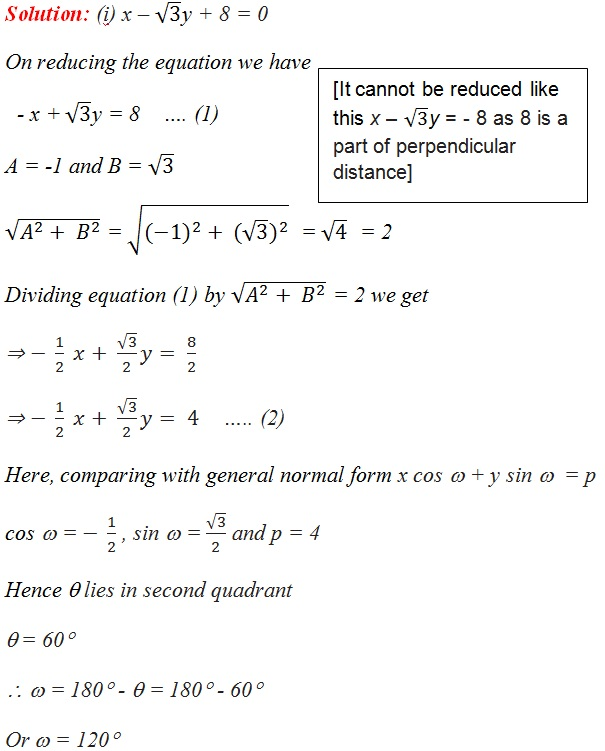Required normal form of the line.

X cos 120° + y sin 120° = 4

Perpendicular distance (p) = 4

And angle between perpendicular and the positive x-axis = 120°

Solution: (ii) y – 2 = 0

On reducing the equation we have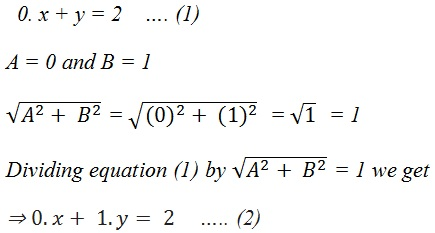Here, comparing with general normal form x cos ω + y sin ω  = p

cos ω =  , sin ω =  and p = 2

Hence point lies on y-axis and θ is in I quadrant.

θ = 90°

∴ ω = 90°

Required normal form of the line.

X cos 90° + y sin 90° = 2

Perpendicular distance (p) = 2

And angle between perpendicular and the positive x-axis = 90°

∴ ω θ

Solution: (iii) x – y = 4

On reducing the equation we have

x - y = 2    …. (1)

A = 1 and B = -1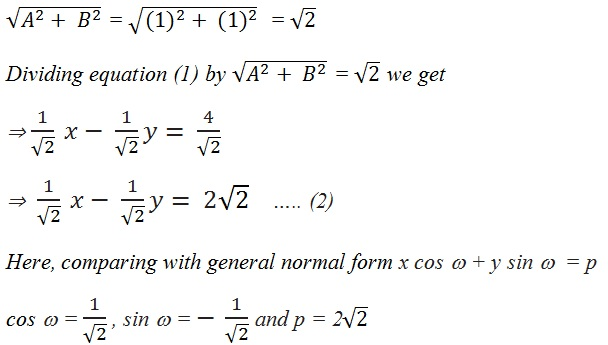Hence ω lies in VI quadrant.

θ = 45°   [ θ is value of angle between 0 - 90°]

ω = 360° - θ = 360° - 45° = 315°

Required normal form of the line.

x cos 315° + y sin 315° = 2

Perpendicular distance (p) = 2

And angle between perpendicular and the positive x-axis = 315°

Q4.  Find the distance of the point (–1, 1) from the line 12(x + 6) = 5(y – 2).

Solution:

Given line is 12(x + 6) = 5(y – 2) which gives

12(x + 6) = 5(y – 2)

12x + 72 = 5y – 10

12x – 5y + 72 + 10 = 0

12x – 5y + 82 = 0     ….. (1)

On comparing equation (1) with general equation of line Ax + By + C = 0, we obtain

A = 12, B = - 5 and C = 82

Now distance from given point (-1, 1) to line 12x – 5y + 82 = 0  given by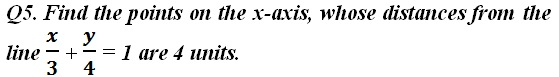Solution: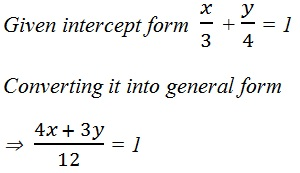4x + 3y = 12

4x + 3y - 12 = 0   …..(1)

Comparing equation (1) with general equation of line Ax + By + C = 0

We obtain, A = 4, B = 3 C = -12

Let the point on x-axis be (a, 0) whose distance from given line is 4 units.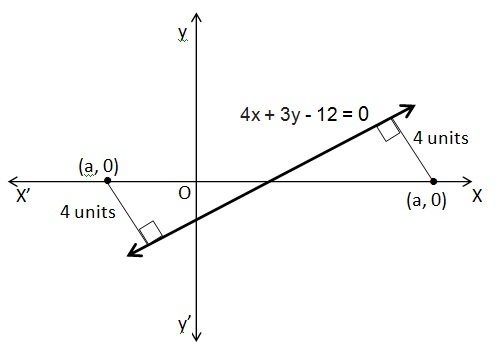Using perpendicular distance formula;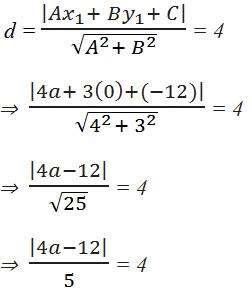±(4a – 12) = 20

Here we take both +ve and –ve signs

4a – 12 = 20   Or  – 4a + 12 = 20

4a = 32   Or  – 4a = 20 – 12

4a = 32   Or  – 4a = 20 – 12 = 8

a = 8 or  a =  – 2

Thus the required point on x-axis are (8, 0) and (-2, 0)

Q6.  Find the distance between parallel lines

(i) 15x + 8y – 34 = 0 and 15x + 8y + 31 = 0

(ii) l (x + y) + p = 0 and l (x + y) – r = 0.

Solution:

(i) 15x + 8y – 34 = 0 and 15x + 8y + 31 = 0

Q12.  Two lines passing through the point (2, 3) intersects each other at an angle of 60°. If slope of one line is 2, find equation of the other line.

Solution:

The slope of one line m1 = 2,

Let the slope of other line be m2.

And the angle between two lines is 60°

θ = 60°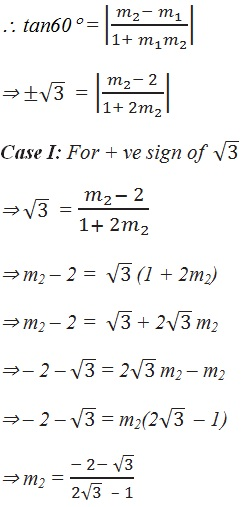Now equation of the given live which passes through (2, 3)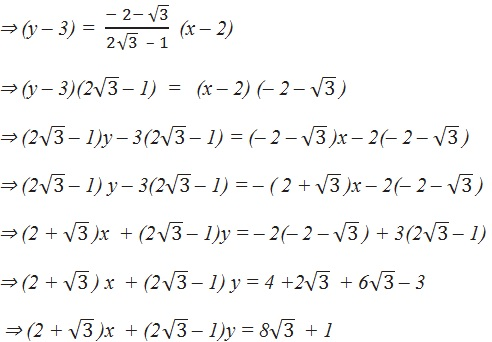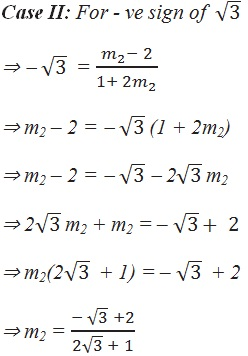Now equation of the given live which passes through (2, 3)

Q13. Find the equation of the right bisector of the line segment joining the points (3, 4) and (–1, 2).

Solution:

Right bisector means perpendicular bisector of given line segment are A(3, 4) and B(-1, 2).

∵ Line bisects AB ​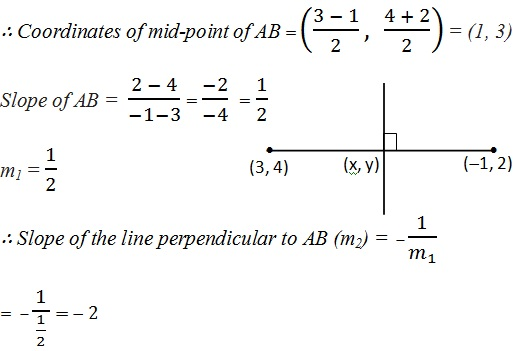The equation of the line passing through (1, 3) and having a slope of –2 is

(y – 3) = –2 (x – 1) y – 3 = –2x + 2

2x + y = 5

Thus, the required equation of the line is 2x + y = 5.

Q14. Find the coordinates of the foot of perpendicular from the point (–1, 3) to the line 3x – 4y – 16 = 0.Solution:

Equation of given line is

3x – 4y – 16 = 0     …………. (1)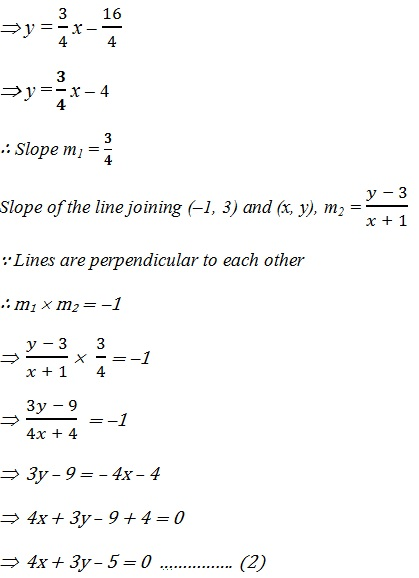Q15. The perpendicular from the origin to the line y = mx + c meets it at the point (–1, 2). Find the values of m and c.

Solution:

Slope of perpendicular line from origin (0, 0) and (–1, 2).Q16. If p and q are the lengths of perpendiculars from the origin to the  lines x cosθ - ysin θ = k cos2θ and x sec θ + y cosec θ  = k, respectively,  prove that p2 + 4q2 = k2.

Solution:

x cos θ – y sinθ = k cos 2θ ………………. (1)

x secθ + y cosec θ = k ………………….… (2)

The perpendicular distance (d) of a line Ax + By + C = 0 from a point (x1, y1) is given by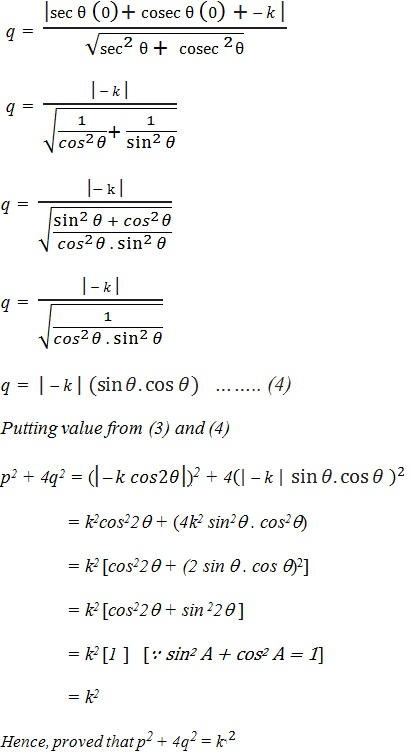Q17. In the triangle ABC with vertices A (2, 3), B (4, –1) and C (1, 2), find the equation and length of altitude from the vertex A.

Solution:

ABC is the triangle which vertices are A (2, 3), B (4, –1) and C (1, 2).

AD is altitude on side BC from vertex A.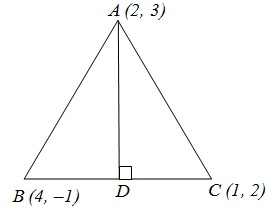(y – 3) = 1 (x – 2)

y – 3 = x – 2

x – y – 2 + 3 = 0

x – y + 1 = 0

The required equation of Altitude is x – y + 1 = 0.

Now equation for line BC where slope is – 1.

(y + 1) = 1 (x – 4)

y + 1  = x + 4

x + y + 1 – 4 = 0

x + y – 3 = 0

Length of AD = Length of the perpendicular from A (2, 3) to BC The equation of BC is x + y – 3 = 0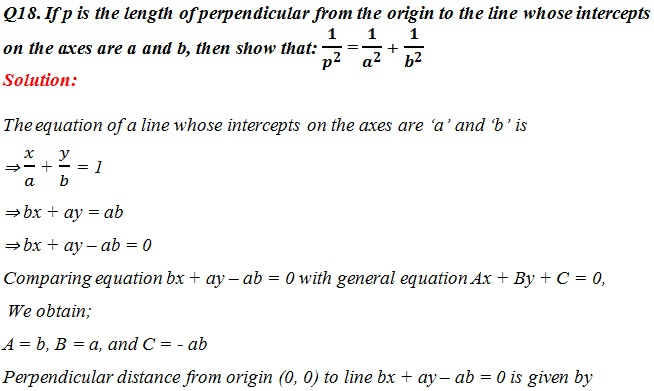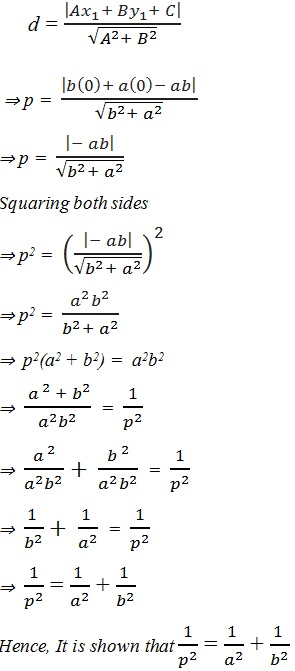##### Other Pages of this Chapter: 10. Straight Lines

Important Study materials for classes 06, 07, 08,09,10, 11 and 12. Like CBSE Notes, Notes for Science, Notes for maths, Notes for Social Science, Notes for Accountancy, Notes for Economics, Notes for political Science, Noes for History, Notes For Bussiness Study, Physical Educations, Sample Papers, Test Papers, Mock Test Papers, Support Materials and Books.Mathematics Class - 11th

NCERT Maths book for CBSE Students.

books

## Study Materials List:

##### Solutions ⇒ Class 11th ⇒ Mathematics
1. Sets
2. Relations and Functions
3. Trigonometric Functions
4. Principle Of Mathematical Induction
5. Complex Numbers and Quadratic Equations
6. Linear Inequalities
7. Permutations and Combinations
8. Binomial Theorem
9. Sequences and Series
10. Straight Lines
11. Conic Sections
12. Introduction to Three Dimensional Geometry
13. Limits and Derivatives
14. Mathematical Reasoning
15. Statistics
16. Probability

New Books